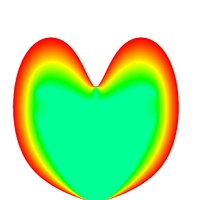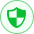MATLAB及科学计算

2位雇主推荐MATLAB及科学计算

# MATLAB及科学计算

2位雇主推荐

`承接MATLAB程序设计，界面设计，c/c++程序设计。主要服务内容为科学计算相关问题，也可以涉及其他方面。承接燃烧传热等问题的CFD模拟和分析。`
`承接MATLAB程序设计，界面设计，c/c++程序设计。主要服务内容为科学计算相关问题，也可以涉及其他方面。承接燃烧传热等问题的CFD模拟和分析。`
• 4.99
综合评分
• 183,968
成交额
• 100%
好评率
• 100%
项目完成率matlab 算法 仿真 程序 c/c++ CFD 燃烧传热
¥ 100.0 /件

• 合作很多次了，专业强，服务态度好，**。合作愉快。很满意，大大超出了预期。

matlab 算法 仿真 程序 c/c++ CFD 燃烧传热 2000.00

2019-04-28

matlab 算法 仿真 程序 c/c++ CFD 燃烧传热 6400.00

2019-01-25
t_1886ghg9iq
matlab 算法 仿真 程序 c/c++ CFD 燃烧传热 3500.00

2018-06-13
t_3384unpb88
matlab 算法 仿真 程序 c/c++ CFD 燃烧传热 4200.00

2018-05-08

matlab 算法 仿真 程序 c/c++ CFD 燃烧传热 2000.00

2018-05-04